# New method to resolve 2π ambiguity in NBV

## Abstract

The Narrow Bandwidth VLBI (NBV) technique is utilized to track spacecrafts in Japanese lunar exploration. However, the problem of 2π ambiguity affects the phase delay of the carrier waves transmitted by satellites. A probabilistic algorithm called coarse search and fine delay search, in combination with a simple ambiguity judgment procedure, is presented in the current paper to resolve the 2π ambiguity. This method is employed to estimate both the delay and the delay rate from the residual phases obtained using NBV. Compared with previous analytic methods, it does not require strict constraint conditions. The ambiguity can be resolved even when the phase variations in the residual phase are 0.2 rad (approximately 11.6 degrees) compared with the less than 4.3 degree phase variations in the analytic method. The method also has the advantage of giving short time variations in the orbital motion of a satellite without ambiguity using NBV.

## 1. Introduction

In the Japanese lunar explorer SELenogical and ENgineering Explorer (SELENE) project, three satellites, including a main orbiter, a relay sub-satellite (Rstar), and a VLBI sub-satellite (Vstar), were launched into polar orbits around the Moon. Using four-way Doppler and differential VLBI techniques, the global map of the lunar gravity field was substantially improved. The measurement of the gravity field, obtained through the orbital motion of a spacecraft, is a powerful method to estimate the inner density structure of the Moon (Iwata et al.,2009).

The VLBI technique has been used in spacecraft tracking since the 1960s. However, one persisting problem is the ambiguity existing in the phases of the carrier waves transmitted by satellites. Therefore, group delay and delay rate have been primarily used (Border et al., 1992). However, the accuracy of group delay is limited to several hundred pico-seconds (ps), which is insufficient for a precise lunar gravity field estimation. To determine the low degree coefficients of the lunar gravity field, the estimation of phase delay with an accuracy of several ps is necessary.

Two radio sources are loaded on Rstar and Vstar, with each transmitting three carriers in the S-band and one carrier in the X-band. In the work of Kono et al. (2003), these sources help address cycle ambiguity using the multi-frequency VLBI (MFV) method (Kono et al., 2003), especially using the same-beam MFV method, when the elongation between two nearby spacecrafts is smaller than the beam width of the ground antenna (Liu et al., 2007; Kikuchi et al., 2009). In their methods, cycle ambiguity was derived analytically. The residual phase equation pairs were deduced individually, relying on strict error conditions, e.g. the error of the correlation phase must be less than 4.3°. Further, the ionospheric fluctuation must be less than 0.23 TECU (1 TECU equals 1016 el/m2). The TEC condition is more difficult for switching VLBI when a traveling ionospheric disturbance occurs in the ionosphere. However, it is addressed through the use of the same-beam method.

The derivation of cycle ambiguity is a critical aspect. Tropospheric fluctuation is a flicker noise (Liu et al., 2005). Therefore, the phase error cannot be reduced in inverse proportion to the integration time, and coherence decreases when the integration time is longer than a few tens of seconds. In the analytic method, the integration time is set to be approximately 1 min to decrease the number of phase variations. However, this duration is unsuitable for precise orbit determination and gravity field recovery.

In the current study, a new delay and delay rate estimation method is presented. The proposed method is based on probability theory and is called coarse search and fine delay search. It has been widely used in conventional VLBI. With this method, cycle ambiguity can be resolved with less stringent conditions in which the phase error of the residual phase is less than 0.2 rad. This condition indicates that the integration time becomes only 1/4 of that of the previous method, and shorter time information of the orbital motion can also be given. The improvement in time resolution in relative positioning is very important for the docking of two satellites and for understanding the dynamics of a medium-sized crater.

## 2. Main Concept

### 2.1 Main principle of coarse search and fine delay search in NBV

Coarse search and fine delay search are described in this section. They are used to obtain the residual group delay and residual delay rate in a conventional VLBI (Rogers, 1970). Only a few frequency components exist in each channel in NBV, and the search procedures between NBV and conventional VLBI are expected to be different (Takahashi et al., 2000; Thornton and Border, 2003). Coarse search is only used to obtain the residual delay rate in the current study, whereas fine delay search is employed to derive the phase delay of radio frequency (RF) carriers. For the n-th channel, the correction function in the coarse search can be expressed as follows,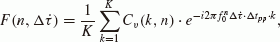(1)

where C υ (k, n) represents the residual phase of channel n, which can be calculated from the time, or frequency, domain. k indicates the kth integration period, and n is the nth channel.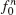is the RF frequency of channel n, Δt pp is the integration period, and pp is defined as a unit of integration time, which is different from that in conventional VLBI wherein pp is defined as a period when all parameters for data analysis are constant. The coarse search is then the arithmetic mean of the absolute value of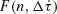through all frequency channels, which can be expressed as follows,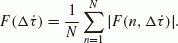(2)

The coarse search is used to find the value ofwhich maximizes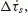. Using the residual delay rate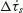, which was obtained using the coarse search, D(n) is defined to establish the following fine delay resolution function,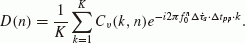(3)

Using D(n), the fine delay resolution function is given as follows,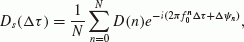(4)

where Δψ n = ψ nx ψ ny , in which ψ nx and ψ ny are the mean values of the phases measured using a phase calibrator for channel n of the sub-station and reference station, respectively. Then, Max [|D s τ)|] corresponds to the final delay Δτfine. Equation (4) is summed from channel 0 to N. Channel 0 always indicates that the phase is 0 at a frequency of 0. This term is not included in the bandwidth synthesis for conventional VLBI. The addition of channel 0 is important because it improves the estimated delay accuracy from group delay to phase delay. However, the total phase is difficult to obtain because of various delay error sources and unknown phases, as discussed in Section 2.2. Equation (4) is generally summed from 1 to N, which can also improve the accuracy to several tens of ps if both frequencies in the S-band and X-band are selected. A higher accuracy can be achieved using same-beam differential VLBI when the effects of the atmosphere, ionosphere, and receivers are almost canceled from the difference in correlation phase, and when channel 0 can be added (Liu et al., 2007; Kikuchi et al., 2009).

### 2.2 Problem of 2π ambiguity

2π ambiguity problems result from the fine delay search procedure, both in conventional VLBI and NBV. The delay obtained from the fine delay search is the slope of phases with respect to frequency, assuming that all fringe phases of each channel can be connected to form a straight line (Fig. 1). The inclination corresponds to the time delay.

However, the phase of each channel (e.g. Channel 4 in Fig. 1) can be validated in multiples of 2π, and this characteristic results in the ambiguity of delay, and fine delay search procedures are sometimes unsuccessful. In a previous study (Kono et al., 2003; Kikuchi et al., 2009), multi-frequency and same-beam differential VLBI technology are introduced to resolve ambiguity, and the correct delay values are obtained with an approximately 1 minute integration. In the current study, the 2π ambiguity problem can also be resolved with a shorter integration time by the coarse search and fine delay search method, and using the total delay and delay rate values predicted at the present epoch as references for judgment, which are derived from the values of the former epoch.

## 3. Simulation Analysis

The data analysis method for same-beam MFV observation of SELENE was adopted in the simulations. The differential residual fringe phase (ΔRFP) of carriers can be simply expressed as follows (Kono et al., 2003; Kikuchi et al., 2009):(5)

where Δϕ n and Δ2ϕ n are the residual and differential residual fringe phases of channel n, s1 and s2 represent the radio sources Rstar and Vstar, Δ2τ(t) and ΔN are the differential residual delays varying with time and the cycle ambiguity, respectively, and σ φn is the phase error which includes the phase noise from the atmosphere and random noise from receivers, among others.

In the simulations, the RF frequency components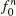(n = 1, 2, 3, 4) consisted of three carrier wave signals in the S-band (2.212, 2.218, and 2.287 GHz) and one in the X-band frequency (8.456 GHz). The differential residual delay was fitted with a five-order polynomial based on a real orbit model. Differential phase noise for same-beam observation was derived from the observed tropospheric phase variations (Liu et al., 2005) assuming different traveling times (4, 5, 8, and 11s). Traveling time is proportional to the elongation between Rstar and Vstar. The differential frequency-dependent ionospheric delay was less than 5 ps in the S-band and less than 0.3 ps in the X-band (Liu et al., 2007), which can be disregarded. The simulation signal used as a received signal was produced by adding random noise generated by random numbers in a computerand the observed tropospheric phase variations. The root mean square (RMS) of the fringe phase errors with a pp integration were set to 18.0 degrees for the S-band and 26.6 degrees for the X-band, which can diminish to 3.1 degrees and 5.2 degrees with 50 s integration, respectively. Coarse search and fine delay search of the simulation signals were carried out to acquire the residual delay and the residual delay rate.

The procedure to derive cycle ambiguity is illustrated as follows. Wrong delay may be determined from the current phase information and also from the former delay and delay rate. Although the resulting ambiguity in the estimation of the X-band phase delay is only 1/(8.456 GHz), a wrong ambiguity is rarely estimated, and a probable wrong ambiguity may be greater than 1/(2.287 GHz). The search function is basically a maximum likelihood estimation because three frequency components exist in the S-band, whereas only one exists in the X-band. Therefore, a stricter condition in phase delay fitting error is required in the S-band. A greater number of wrong ambiguities are estimated in the determination of the S-band phase delay. The corresponding difference between a correct and a wrong ambiguity is 1/(2.287 GHz). The predicted delay is within an accuracy of 1 ns using range and range rate measurement and the loose phase error condition needed for the correct estimation. Therefore, the wrong ambiguity is poorly estimated, resulting in a large gap in delay. A priori estimate values, which can provide an approximately correct criterion for judgment, were simply calculated as follows,(6)

where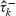and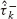are a priori estimates of the delay and delay rate at epoch k,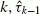and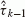are a posteriori estimate values at epoch k − 1, and Δt is the integration time.

The flow chart of ambiguity judgment for real delay and delay rate determination is shown in Fig. 2.

First, the total integration time must be roughly determined. Based on the capability to acquire delay rate correctly, the threshold of b is set at approximately several tens of ps/s. Second, the threshold of a is expected to be between 0.118 and 0.437 ns, with the former corresponding to 1 ambiguity for the X-band carrier and the latter to 1 ambiguity for the S-band carrier. If a wrong ambiguity is detected, the quantity of the ambiguity will be counted with 1 ambiguity corresponding to the carrier as a unit. The delay value is then shifted with the ambiguities, and the results are considered as a posteriori estimates. The a priori estimates for the next epoch are revised using Eq. (6), and the process is repeated.

However, the estimation method is dependent on the accuracy of the initial a priori estimates. When the first a priori estimate values significantly deviate from the true values over the threshold, such as 0.2 ns for delay and several tens of ps/s for the delay rate, the results are no longer valid. The first correct delay needs to be estimated using long-term integration so that the phase error will be sufficiently small to resolve 2π ambiguity. However, such an integration time is extremely long so the phase variation exceeds π in some cases.

In this case, the estimated delay is incorrect, so the carrier signal cannot be correctly detected. Assuming that both the phase variations attributable to the atmosphere, and the random noise from receivers, are distributed as Gaussian functions, a frequency distribution table with respect to the ambiguity can be generated by applying the search procedures several times in a given period. The frequency at the correct ambiguity would then be at a maximum in the table. If the first correct delay value is evaluated in this method, and the same procedure is repeated during the observation period, appropriate delay times through the whole observation period can be estimated accordingly.

## 4. Simulation Results

Figures 3(a), 3(b), and 3(c) show the estimation errors with respect to the RMS of the instant phase errors of four carriers. The phase errors were calculated after each total integration time. The unit of delay rate errors is ps/s, whereas that of delay error is 1/8.456 ns. Figure 3(a) indicates that, even when the integration time is 5 s and the phase errors are larger than 0.2 rad, a possibility for the search method to resolve the ambiguity still exists, which is the primary advantage of this method. In Fig. 3(b), increasing the integration time decreases the probability of deriving a wrong ambiguity. However, this method fails to acquire an adequate amount of scientific data. Meanwhile, as shown in Fig. 3(c), if the predicted value is used to determine whether a wrong ambiguity occurs, the number of cycle ambiguities of ΔRFP can be resolved in most cases.

In Fig. 4, the tropospheric phase noise samples with different traveling times were used. According to the process used in Fig. 3, three groups of simulations were conducted using different samples. The number of possibilities of resolving the cycle ambiguity were counted, based on different phase error conditions. The results of the 5 and 15 s integration times without an ambiguity judgment were combined in one group. The filled bars in Fig. 4 correspond to the results with an ambiguity judgment for 5 s integration. The finding shows that the ambiguities can be resolved when the phase errors are less than 0.2 rad. When the phase errors exceed 0.2 rad, Fig. 3(c) shows that the ambiguity can still be resolved using an ambiguity judgment. This finding is primarily attributed to an accurate a priori estimated residual delay and residual delay rate in Eq. (6) acquired in the case of small phase errors. However, as shown in Fig. 3(a), when the phase errors exceed 0.2 rad, the possibility of searching a correct ambiguity is reduced to half, or even less. Distinguishing the correct ambiguity from wrong ambiguities in the frequency distribution table, which is essential for determining the first correct delay and to evaluate the correct delay within the observation period, will be difficult in practice. In this case, the ambiguity judgment is useless.

In Fig. 4, when the phase errors are larger than 0.2 rad, the possibility of resolving the ambiguity using an ambiguity judgment must be set as a conditional probability, which is equal to the product of the possibilities of searching a correct ambiguity and resolving ambiguity using an ambiguity judgment, assuming a correct a priori delay and delay rate. A value of 0.2 rad is the upper limit of the phase error in a conservative estimate to resolve ambiguity, with a corresponding integration time 1/4 of that needed in the previous method. Therefore, a greater amount of information on orbital motion can be obtained by applying this condition.

## 5. Discussion and Conclusion

To resolve 2π ambiguity, and to estimate the phase delay of an RF carrier, phase errors resulting from the atmosphere and receiver noise, among others, should be controlled to a low level. Switching VLBI, especially same-beam VLBI, can decrease a large portion of the errors and can finally provide the conditions for completely resolving ambiguity. Still, the integration time should be adequately long to enable the same-beam method to reduce phase errors and to resolve the ambiguity using the analytic solutions employed in previous work.

In the current study, a probabilistic algorithm, which can be used to obtain correct results under comparatively less stringent conditions, is presented. To realize this goal, there is a need to ensure first the estimation accuracy of the initial values. This estimation accuracy can also be solved using probabilistic algorithms to obtain the frequency distribution of ambiguity and to determine the first correct delay. The current study proposes another method for resolving ambiguity, in which shorter period information on orbital motion can be obtained because of the shorter integration time. Further, the computations, including coarse search and fine delay search and the ambiguity judgment procedure, can be easily performed using a personal computer. The application of a Kalman filter for delay and delay rate estimation, and the use of real data from SELENE, are expected to be done in our future work.

## References

• Border, J. S., W. M. Folkner, R. D. Kahn, and K. S. Zukor, Precise tracking of the Magellan and Pioneer Venus Orbiters by same-beam interferometry, part I: Data accuracy analisis, TDA Progress Report, 42–110, Aug. 15, 1992.

• Iwata, T., H. Minamino, T. Sasaki, M. Ogawa, N. Namiki, H. Hanada, H. Noda, K. Matsumoto, T. Imamura, Y. Ishihara, S. Tsuruta, K. Asari, Q. Liu, F. Kikuchi, S. Goosens, T. Ishikawa, N. Kawano, and T. Takano, Mission outline of selenodesy by KAGUYA(SELENE) and development and on-orbit properties of sub-satellites: Okina and Ouna (Rstar and Vstar), J. Geod. Soc. Jpn., 55(2), 135–150, 2009.

• Kikuchi, F., Q. Liu, H. Hanada, N. Kawano, K. Matsumoto, T. Iwata, S. Goossens, K. Asari, Y. Ishihara, S. Tsuruta, T. Ishikawa, H. Noda, N. Namiki, N. Petrova, Y. Harada, J. Ping, and S. Sasaki, Picosecond accuracy VLBI of the two subsatellites of SELENE (KAGUYA) using multifrequency and same beam methods, Radio Sci., 44, RS2008, 2009.

• Kono, Y., H. Hanada, J. Ping, Y. Fuzuzaki, and N. Kawano, Precise positioning of spacecrafts by multi-frequency VLBI, Earth Planets Space, 55, 581–589, 2003.

• Liu, Q., M. Nishio, K. Yamamura, T. Miyazaki, M. Hirata, T. Suzuyama, S. Kuji, K. Iwadate, O. Kameya, and N. Kawano, Statistical characteristics of atmospheric phase fluctuations observed by a VLBI using a beacon wave from a geostationary satellite, IEEE Trans. Antennas Propagation, 53(4), 1519–1527, 2005.

• Liu, Q., F. Kikuchi, K. Matsumoto, K. Asari, S. Tsuruta, J. Ping, H. Hanada, and N. Kawano, Error analysis of same-beam differential VLBI technique using two SELENE satellites, Adv. Space Res., 40(1), 51–57, 2007.

• Rogers, A. E. E., Very-long baseline interferometry with large effective bandwidth for phase-delay measurements, Radio Sci., 5, 1239, 1970.

• Takahashi, F., T. Kondo, Y. Takahashi, and Y. Koyama, Very Long Baseline Interferometer, IOS Press, 2000.

• Thornton, C. L. and H. S. Border, Radiometric tracking techniques for deep-space navigation, in JPL Deep Space Communication and Navigation Series, edited by Yuen, J. H., 85 pp., Wiley and Sons Inc., US, 2003.

## Acknowledgements

The authors wish to thank the editor and two anonymous referees for their comments. The current work was financially supported by the National Natural Science Foundation of China (No. 10973033) and the Shanghai Natural Science Foundation (09ZR1437300).

## Author information

Authors

### Corresponding author

Correspondence to Wu Jiang.

## Rights and permissions

The images or other third party material in this article are included in the article’s Creative Commons licence, unless indicated otherwise in a credit line to the material. If material is not included in the article’s Creative Commons licence and your intended use is not permitted by statutory regulation or exceeds the permitted use, you will need to obtain permission directly from the copyright holder.

To view a copy of this licence, visit https://creativecommons.org/licenses/by/4.0/.

Reprints and Permissions

Jiang, W., Kawano, N., Kikuchi, F. et al. New method to resolve 2π ambiguity in NBV. Earth Planet Sp 64, 37–41 (2012). https://doi.org/10.5047/eps.2011.08.008

• Revised:

• Accepted:

• Published:

• Issue Date:

• DOI: https://doi.org/10.5047/eps.2011.08.008

### Key words

• VLBI
• 2π/cycle ambiguity
• coarse search
• fine delay search
• delay and delay rate
• NBV